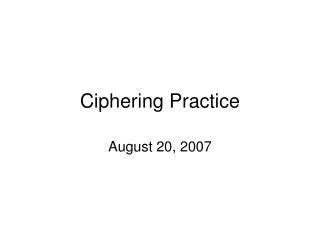DownloadDownload PresentationCiphering Practice

# Ciphering Practice

Download Presentation## Ciphering Practice

- - - - - - - - - - - - - - - - - - - - - - - - - - - E N D - - - - - - - - - - - - - - - - - - - - - - - - - - -
##### Presentation Transcript

1. Ciphering Practice August 20, 2007

2. If , what are the values of k, such that ?

3. If you ask Batman’s nemesis, Catwoman, how many cats she has, she answers with a riddle: “Five-sixths of my cats plus seven.” How many cats does Catwoman have?

4. In right triangle ABC, with right angle ACB, AC=8 inches and BC=6 inches. Point X is on AC, equidistant from A and B. Find CX

5. When Billy turned 14 years old, his father was 42 years old, which was three times Billy’s age at the time. Today is Billy’s birthday, and Billy’s father is now twice as old as Billy. How old is Billy?

6. A parallelogram has vertices at , , , and , where m and n are both positive numbers. How many units are in the length of the longest diagonal of the parallelogram?

7. An ice-cream cone, which is 8cm in diameter, is packed full of ice cream; and a generous hemisphere of ice cream is placed on top. If the volume of the cone is the same as the volume of the ice cream on top of the cone, find the height of the cone.

8. In a three-digit number, the hundreds digit is greater than 5, the tens digit is greater than 4 but less than 8, and the units digit is the smallest prime number. How many three digit numbers satisfy all these conditions?

9. Let a be the number of digits of 22001, and let b be the number of digits of 52001. Compute .

10. How many full seven-day weeks are in seven consecutive years? Assume that the first day of the first year is the first day of the week?